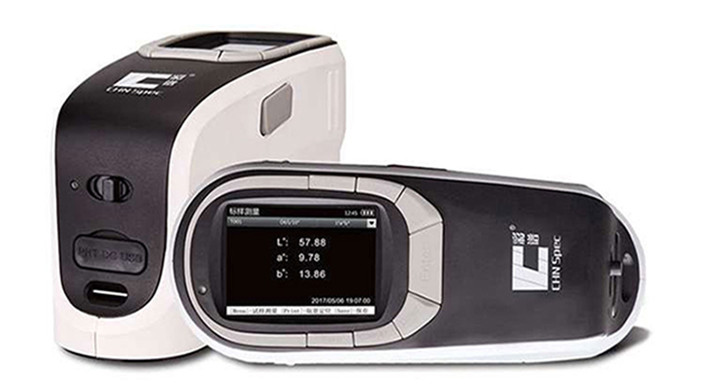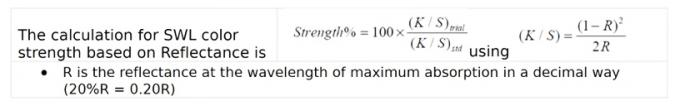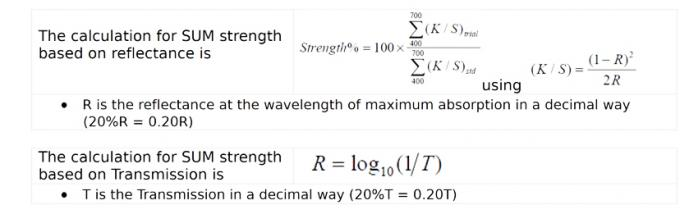Contact Person : Ivy Zhang

Phone Number : +86 18768499725

WhatsApp : +8618768499725

## Opacity and Color Strength Measurement by Color Spectrophotometer

June 10, 2020Portable color spectrophotometers made by CHNSpec Tech can measure both opacity and strength on instrument. We will introduce these two parameter now.

Part 1. Opacity Introduction

Opacity is a measure for hiding power:

Opacity% = Y (black background) / Y (white background) * 100%The measurement of opacity is generally used in plastic products, spray coating, glass, transparent or translucent products and other industries.

Part 2. Color Strength, Apparent Strength and Integrated Strength IntroductionColor Strength SWL

This strength is also known as the chromatic color strength. It describes the ratio based on the (K/S-value) of the Sample in relation to the (K/S-value) of the Standard at a single wavelength and will be expressed in percent. This calculation typically is meaningful, if it will be made at the wavelength of maximum absorption (lowest reflectance). In daily application often it will be made at other wavelength, but results have to be evaluated very carefully. If standard and sample have different wavelengths of maximum of absorption this method will not deliver correct results.Color Strength SUM (DIN55986)

This strength method is sometimes listed as apparent strength.

The % Color strength SUM represents the ratio of (K/S) data between ample and standard at all visual wavelength (400-700nm) and will be expressed in % . The selection of different illuminant observer condition has no influence on the result.Interpretation

Percent color strength > 100 = sample is more strong in color than the standard.

Percent color strength < 100 = sample is weaker in color than the standard.

Percent color strength = 100 = sample and standard have the same color strength.

Color Strength WSUM

This strength method is sometimes listed as integrated strength.

The strength WSUM represents the ration of (K/S) data multiplied by the sum of weighted observer/illumination at all wavelengths for the sample in relation to the standard. It will be expressed in percent.The result is illuminant/observer depending.Interpretation

Percent color strength > 100 = sample is more strong in color than the standard.

Percent color strength < 100 = sample is weaker in color than the standard.

Percent color strength = 100 = sample and standard have the same color strength.